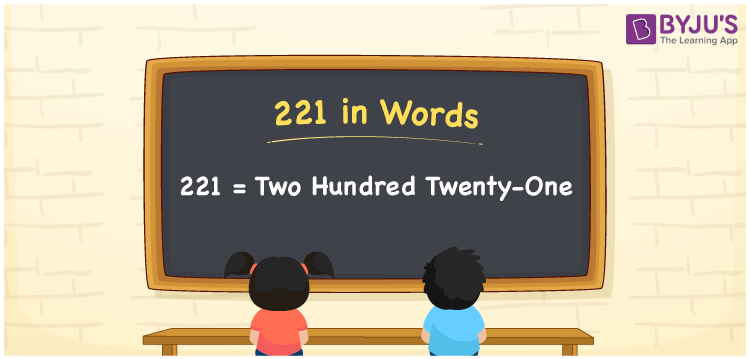# 221 in Words

221 in words can be written as Two Hundred Twenty-One. If you construct a triangle with an angle of 221 degrees, then you can say that “I have to construct a triangle with an angle Two Hundred Twenty-One degrees”. The place value chart of 221 can be used to write the numbers using the English alphabet. Therefore, 221 can be read as “Two Hundred Twenty-One” in words.

 221 in words Two Hundred Twenty-One Two Hundred Twenty-One in Numbers 221

## 221 in English Words## How to Write 221 in Words?

Three digits are present in 221, whose place value can be understood using the chart given below.

 Hundreds Tens Ones 2 2 1

221 in expanded form is:

2 × Hundred + 2 × Ten + 1 × One

= 2 × 100 + 2 × 10 + 1 × 1

= 200 + 20 + 1

= 221

= Two Hundred Twenty-One

Therefore, 221 in words is written as Two Hundred Twenty-One.

221 is a natural number that precedes 222 and succeeds 220.

221 in words – Two Hundred Twenty-One

Is 221 an odd number? – Yes

Is 221 an even number? – No

Is 221 a perfect square number? – No

Is 221 a perfect cube number? – No

Is 221 a prime number? – No

Is 221 a composite number? – Yes

## Frequently Asked Questions on 221 in Words

Q1

### Write 221 in words.

221 can be written in words as “Two Hundred Twenty-One”.
Q2

### How do you write Two Hundred Twenty-One in numbers?

Two Hundred Twenty-One can be written in numbers as 221.
Q3

### Is 221 an even number?

No, 221 is not an even number as it is not completely divisible by 2.